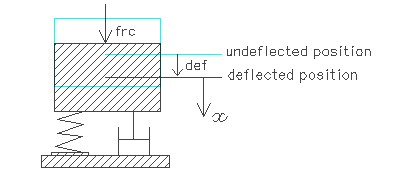FCVSTP,frq,dmr,def,sel
Forced vibration under a constant force with damping

frq         natural vibration frequency in KHz
dmr       damping ratio
def        displacement from stable position due to the constant force in µm
sel         number denoting the selected result.
Use 1 for damped frequency of vibration, 2 for settling time and 3 for period of vibrationNotes

The natural frequency of vibration of structures estimated under Vibration > Free vibration assumes that damping is not present and no external force is acting on the structure. In reality, some form of damping and external forces are present in many MEMS devices. In the case of a capacitive accelerometer, due to the electrostatic force generated by the supply voltage, the inertial mass would move to a new stable position. When it moves, it will overshoot the new deflected position. Since it is an unbalanced state it will move back to the undeflected position and in this way it will oscillate about the new deflected position.  Damping will reduce the oscillation amplitude with every cycle and finally bring the structure to the new stable position denoted by the deflected position 'def ' above.

This design interface can be used to estimate the vibration frequency, settling time and period of vibration considering the damping and the steady force involved. The damping has significant effect on the vibration frequency depending on the damping ratio. The free vibration frequency is as estimated for the various structures under Vibration > Free vibration. If the damping ratio for air damping is not available it could be estimated using the design forms under Vibration > Damping for a given air gap distance. If the spring constant is k then the deflection 'def' is equal to frc/k. If spring constant is not known it can be calculated under Mechanics > Structures. For a parallel plate actuator, the balanced displaced position can be found under Actuation > Electrostatic > Parallel plate.

The vibration frequency is estimated for the under damped case only as there is no oscillation of displacement for the over damped and critically damped cases. For the critically damped system, the damping ratio is equal to one while for the over damped case it is greater than one and for under damped it is less than one. The period of vibration is the inverse of frequency in microseconds. Settling time is the time needed to bring the vibrating mass to a near stable position. Settling time is calculated as the time required to bring the displacement down to 0.1% of the deflected position 'def'. This will be smaller for a critically damped part.

The plot shows displacement of the system over a time scale in microseconds for under damped, critically damped and over damped vibration based on the given damping ratio. For a critically damped system, the displaced mass will settle down to the new steady state position immediately while for the over damped case it will take longer. The displacement is plotted upto three time periods in the time scale. Using the crosshair tool, the displacement at any point after time t = 0 can be estimated from the graph. Settling time is the time in the X axis when the graph nearly flattens out at x = def. The undamped case can be created by setting the damping ratio equal to zero.

Assumptions

-The external force is constant in magnitude and direction and does not change with time.
-For air damping, the damping ratio should correspond to that particular mode of vibration.